# Pipe and Cistern Questions

Pipe and Cistern Questions for SSC CGL, CHSL, CPO, JE, Steno, MTS and all Competitive Exams. Free online practice set for English Medium students for the preparation of upcoming Staff Selection Commission Exams.

## Pipe and Cistern Questions : Practice Set

### Questions based on the concept of pipe and cistern

Question 1:
Two pipes A and B can fill a tank in 20 minutes and 30 minutes respectively. If both pipes are opened together, the time taken to fill the tank is :
(1) 50 minutes
(2) 12 minutes
(3) 25 minutes
(4) 15 minutes

(2) 12 minutes
Part of tank filled in one minute
=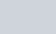$\dfrac{1}{20} + \dfrac{1}{30}$
Required time=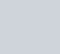$\dfrac{1}{\dfrac{1}{20} + \dfrac{1}{30}}$ =12 minutes

Question 2:
Two pipes A and B can separately fill a cistern in 60 minutes and 75 minutes respectively. There is a third pipe in the bottom of the cistern to empty it. If all the three pipes are simultaneously opened, then the cistern is full in 50 minutes. In how much time the third pipe alone can empty the cistern?
(1) 110 minutes
(2) 100 minutes
(3) 120 minutes
(4) 90 minutes

(2) 100 minutes
Let the third pipe empty the cistern in x minutes. Part of cistern filled in 1 minute when all three pipes are opened simultaneously
=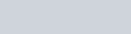$\dfrac{1}{60} + \dfrac{1}{75} -\dfrac{1}{x} = \dfrac{1}{50}$
x=100 minutes

Question 3:
A tap can fill a cistern in 8 hours and another tap can empty it in 16 hours. If both the taps are open, the time (in hours) taken to fill the tank will be :
(1) 8
(2) 10
(3) 16
(4) 24

(3) 16
Part of the cistern filled in 1 hour =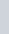$\dfrac{1}{8}$
Part of the cistern emptied in 1 hour=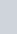$\dfrac{1}{16}$
When both the taps are opened simultaneously, part of cistern filled in 1 hour:
=$\dfrac{1}{8}$$\dfrac{1}{16}$ =$\dfrac{1}{16}$
Hence the cistern will be filled in 16 hours.

Question 4:
12 pumps working 6 hours a day can empty a completely filled reservoir in 15 days. How many such pumps working 9 hours a day will empty the same reservoir in 12 days ?
(1) 15
(2) 9
(3) 10
(4) 12

(3) 10
9 × 12 × x = 6 × 12 × 15
x=10.

Question 5:
A tank can be filled by pipe A in 2 hours and pipe B in 6 hours. At 10 A.M. pipe A was opened. At what time will the tank be filled if pipe B is opened at 11 A.M.?
(1) 12.45 A.M.
(2) 5 P.M
(3) 11.45 A.M.
(4) 12 P.M

(3) 11.45 A.M.
Part of the tank filled in 1 hour by pipe A =$\frac{1}{2}$
Part of the tank filled by both pipes in1 hour =$\frac{1}{2}$ +$\frac{1}{6}$ =$\frac{2}{3}$
So, Time taken to fill$\dfrac{2}{3}$ part = 60 minutes
Time taken to fill$\dfrac{1}{2}$ part = 45 minutes
The tank will be filleld at 11:45 A.M

Question 6:
Two pipes, P and Q, together can fill a cistern in 20 minutes and P alone can in 30 minutes. Then Q alone can fill the cistern in
(1) 62 minutes
(2) 60 minutes
(3) 61 minutes
(4) 51 minutes

(2) 60 minutes
Part of the cistern filled by pipe Q in 1 minute
=$\dfrac{1}{20} - \dfrac{1}{30}$
=$\dfrac{1}{60}$
Required time = 60 minutes

Question 7 :
If two pipes function simultaneously, a tank is filled in 12 hours. One pipe fills the tank 10 hours faster than the other. How many hours does the faster pipe alone take to fill the tank?
(1) 20 hrs
(2) 18 hrs
(3) 15 hrs
(4) 12 hrs

(1) 20 hrs
If the slower pipe fills the tank in x hours, then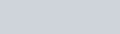$\dfrac{1}{x} - \dfrac{1}{x-10} = \dfrac{1}{12}$
(x-4)(x-30)=0
x=30
30-10=20 hours

Question 8:
A cistern is provided with two pipes A and B. A can fill it in 20 minutes and B can empty it in 30 minutes. If A and B be kept open alternately for one minute each, how soon will the cistern be filled ?
(1) 121 minutes
(2) 110 minutes
(3) 115 minutes
(4) 120 minutes

(3) 115 minutes
Part of the tank filled in first two minutes
=$\dfrac{1}{20} - \dfrac{1}{30}$
=$\dfrac{1}{60}$
Part of tank filled in 114 minutes$\dfrac{57}{60}$ =$\dfrac{19}{20}$
The remaining part will be filled in 115 minutes.

Question 9:
A pipe can fill a tank in x hours and another can empty it in y hours. They can together fill it in (y > x)
(1)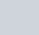$\dfrac{xy}{y-x}$
(2) x-y
(3) y-x
(4)$\dfrac{xy}{x-y}$

When both pipes are opened simultaneously, part of the tank filled in 1 hour
=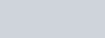$\dfrac{1}{x} - \dfrac{1}{y} = \dfrac{y-x}{xy}$
Required time =$\dfrac{xy}{y-x}$

### Questions based on fractions/part of filled tank :

Question 10:
If$\dfrac{1}{3}$ of a tank holds 80 litres of water, then the quantity of water that$\frac{1}{2}$ tank holds is :
(1) 240 litres
(2) 120 litres
(3)$\dfrac{80}{3}$ litres
(4) 100 litres

(2) 120 litres
Let the capacity of the tank be x litres then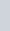$\dfrac{x}{3}$= 80
x = 240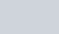$\dfrac{x}{2}= \dfrac{240}{2}$ = 120 litres

Question 11:$\dfrac{3}{4}$ part of a tank is full of water. When 30 litres of water is taken out, the tank becomes empty. The capacity of the tank is
(1) 36 litres
(2) 42 litres
(3) 40 litres
(4) 38 litres

(3) 40 litres
Let the capacity of the tank be x litres.
According to the question,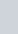$\dfrac{3x}{4}$ = 30
x=40

Question 12:
There are two pumps to fill a tank with water. First pump can fill the empty tank in 8 hours, while the second in 10 hours. If both the pumps are opened at the same time and kept open for 4 hours, the part of tank that will be filled up is :
(1)$\dfrac{9}{10}$
(2)$\dfrac{1}{10}$
(3)$\dfrac{2}{5}$
(4)$\dfrac{1}{5}$

Part of the tank filled in an hour by both pumps
=$\dfrac{1}{8}$ +$\dfrac{1}{10}$
=$\dfrac{9}{40}$
Part of tank filled in 4 hour=$\dfrac{9}{10}$

### Questions based on taps are opened alternatively or a leak:

Question 13:
A pump can fill a tank with water in 2 hours. Because of a leak in the tank it was taking 2$\dfrac{1}{3}$ hours to fill the tank. The leak can drain all the water off the tank in :
(1) 8 hours
(2) 7 hours
(3) 4$\dfrac{1}{3}$ hours
(4) 14 hours

(4) 14 hours
Work done in 1 hour by the filling pump =$\dfrac{1}{2}$
Work done in 1 hour by the leak and the filling pump =$\dfrac{3}{7}$
Work done by the leak in 1 hour=$\dfrac{1}{2}$$\dfrac{3}{7}$ =$\dfrac{1}{14}$
Hence, the leak can empty the tank in 14 hours.

Question 14:
A tap can fill a tank in 6 hours. After half the tank is filled, three more similar taps are opened. What is the total time taken to fill the tank completely ?
(1) 4 hours
(2) 4 hours 15 minutes
(3) 3 hours 15 minutes
(4) 3 hours 45 minutes

(4) 3 hours 45 minutes
A tap can fill the tank in 6 hours. In filling the tank to its half, time required = 3 hours.
Remaining part =$\dfrac{1}{2}$
1 tap takes 6 hours to fill the tank
Time taken by 4 taps take to fill$\dfrac{1}{2}$ of the tank =$\dfrac {3}{4}$ hour
Total time = 3$\dfrac {3}{4}$ hours.

Question 15:
A tank can be filled by two pipes in 20 minutes and 30 minutes respectively. When the tank was empty, the two pipes were opened. After some time, the first pipe was stopped and
the tank was filled in 18 minutes. After how much time of the start was the first pipe stopped?
(1) 5 minutes
(2) 8 minutes
(3) 10 minutes
(4) 12 minutes

(2) 8 minutes
Let the first pipe be closed after x minutes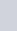$\dfrac{x}{20}$ +$\dfrac{18}{30}$= 1
x= 8 minutes

Question 16:
A tap takes 36 hours extra to fill a tank due to a leakage equivalent to half of its inflow. The inflow can fill the tank in how many hours ?
(1) 36 hrs
(2) 24 hrs
(3) 30 hrs
(4) 18 hrs

(4) 18 hrs$\frac{1}{x}$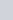$\frac{1}{2x}$ =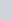$\frac{1}{36}$
x= 18

Question 17:
A cistern is normally filled in 8 hours but takes another 2 hours longer to fill because of a leak in its bottom. If the cistern is full, the leak will empty it in :
(1) 16 hours
(2) 20 hours
(3) 25 hours
(4) 40 hours

(4) 40 hours
Part emptied by the leak in 1 hour
=$\frac{1}{8}$$\frac{1}{10}$
=$\frac{1}{40}$
Time = 40 hours

Question 18:
A pipe can fill a cistern in 9 hours. Due to a leak in its bottom, the cistern fills up in 10 hours. If the cistern is full, in how much time will it be emptied by the leak ?
(1) 70 hours
(2) 80 hours
(3) 90 hours
(4) 100 hours

(3) 90 hours
Part of the tank emptied by the leak in 1 hour
=$\frac{1}{9}$$\frac{1}{10}$
=$\frac{1}{90}$
Time= 90 hours

Question 19:
A tank has two pipes. The first pipe can fill it in 4 hours and the second can empty it in 16 hours. If two pipes be opened together at a time, then the tank will be filled in
(1) 5$\frac{1}{2}$ hours
(2) 10 hours
(3) 6 hours
(4) 5$\frac{1}{3}$ hours

(4) 5$\frac{1}{3}$ hours
Part of tank filled by both the pipes in 1 hour =$\frac{1}{4}$$\frac{1}{16}$
=$\frac{3}{16}$
Required time= 5$\frac{1}{3}$ hours

Question 20:
A water tap fills a tub in ‘p’ hours and a sink at the bottom empties it in ‘q’ hours. If p < q and both tap and sink are open, the tank is filled in ‘r’ hours; then
(1)$\dfrac{1}{r}$=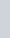$\dfrac{1}{p}$ +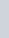$\dfrac{1}{q}$
(2)$\dfrac{1}{r}$=$\dfrac{1}{p}$$\dfrac{1}{q}$
(3) r=p+q
(4) r=p-q

(2)$\dfrac{1}{r}$=$\dfrac{1}{p}$$\dfrac{1}{q}$
P < q
On opening pipe and sink together, Part of the tub filled in 1 hour =$\dfrac{1}{p}$$\dfrac{1}{q}$$\dfrac{1}{r}$=$\dfrac{1}{p}$$\dfrac{1}{q}$

## Miscellaneous Questions :

Question 21:
A pipe can empty a tank in 40 minutes. A second pipe with diameter twice as much as that of the first is also attached with the tank to empty it. The two together can empty the tank in :
(1) 8 minutes
(2) 13$\frac{1}{3}$ minutes
(3) 30 minutes
(4) 38 minutes

(1) 8 minutes
Here, the diameter of the second pipe is twice that of first pipe.
Volume of water emptied by the second pipe will be 4 times to that of first pipe.
Hence, time taken will be$\frac{1}{4}$ of the first pipe.
Second pipe will empty the tank in$\frac{1}{4}$ 40 = 10 minutes
When both the pipes are open, the part of the tank emptied in 1 minute =$\frac{1}{40}$ +$\frac{1}{10}$
=$\frac{1}{8}$
Hence, the tank will be emptied in 8 minutes.

Question 22:
A Boy and girl together fill a cistern with water. The boy pours 4 litres of water every 3 minutes and the girl pours 3 litres every 4 minutes. How much time will it take to fill 100 litres of water in the cistern ?
(1) 36 minutes
(2) 42 minutes
(3) 48 minutes
(4) 44 minutes

(3) 48 minutes
Water filled by the boy and girl in one minute
=$\dfrac{4}{3}$ +$\dfrac{3}{4}$
=$\dfrac{25}{12}$ litres
Time taken to fill 100 litres
=\$latex \dfrac{25}{12} 100 = 48 minutes

Question 23:
Which of these pipes will empty a pool the fastest?
(i) One pipe of diameter 60 cm
(ii) Two pipes of diameter 30 cm each
(iii) Three pipes of diameter 20 cm each
(1) (i)
(2) (iii)7
(3) (ii)
(4) None of these

(1) (i)
If the flow of water per unit time be x km, then
V = p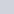$r^2$h [Q Pipe is in cylinderical shape]
Greater the radius, larger thecapacity of pipe
Radius is greatest in (i) i.e. 30cm.
Hence, pipe with 60 cm diameter will empty the pool fastest.

Question 24:
A tap drips at a rate of one drop/sec. 600 drops make 100ml. The number of litres wasted in 300 days is
(1) 4320000
(2) 432000
(3) 43200
(4) 4320

(4) 4320
300 days = (300 × 24) hours
= (300 × 24 × 60 × 60) seconds
Number of drops
= 300 × 24 × 60 × 60
600 drops = 100 ml.
= 300 × 24 × 60 × 60 drops
= 4320 litre

Question 25:
Having the same capacity 9 taps fill up a water tank in 20 minutes. How many taps of the same capacity are required to fill up the same water tank in 15 minutes ?
(1) 10
(2) 12
(3) 15
(4) 18